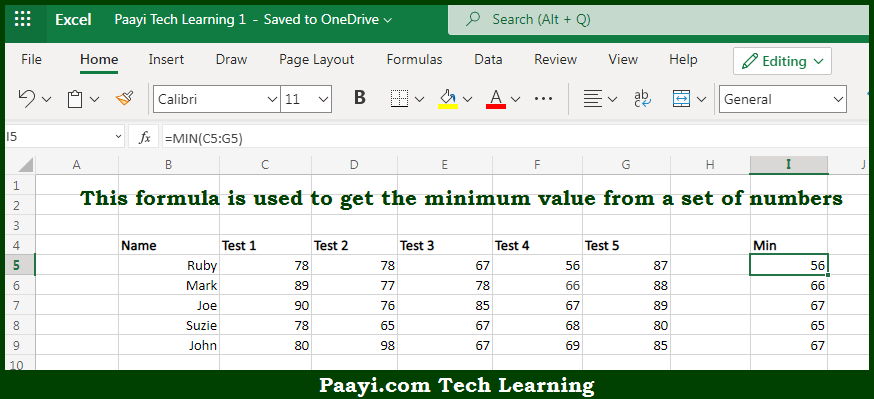# Learn How to Get Minimum Value in Microsoft Excel

Written by | 0 Comments | 328 Views

In this article, you will learn how to evaluate things with MIN and MAX functions in Microsoft Excel using a single/combination(s) of functions. You will also know to Get Minimum Value and see the generic formula.

Learn How to Get Minimum Value in Microsoft Excel

The main purpose of this formula is to get the minimum value from a set of numbers. Here we will learn how to get the minimum value provided in the given data range in the workbook in Microsoft Excel. That implies, with the help of a formula based on the MIN function you can able to get the minimum value from a set of numbers. So, with the help of this formula, you can able to get the minimum value provided in the given data range in the workbook in Microsoft Excel.

General Formula to Get Minimum Value

=MIN(range)

The Explanation to Get Minimum ValueSo we know that with the help of the given formula above you can able to get the minimum value from a set of numbers. Here we will learn how to get the minimum value provided in the given data range in the workbook in Microsoft Excel. As we know that  MIN function accepts one or more arguments. These arguments can be a mix of constants, cell references, and ranges. It should be noted that the MIN function is used to return the maximum value in the data provided. However, the text values and empty cells are ignored. So now you have learned how to get the minimum value provided in the given data range in the workbook in Microsoft Excel.